# Lecture 3 Creating Mfiles Programming tools Inputoutput assigngraphdisplay

• Slides: 17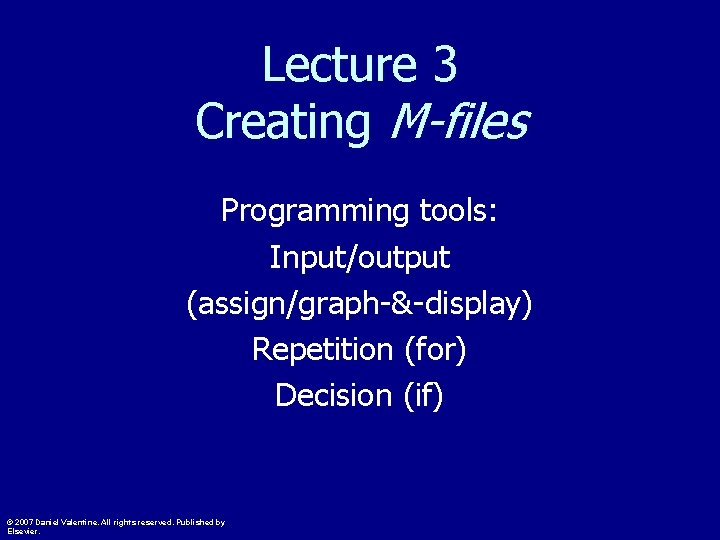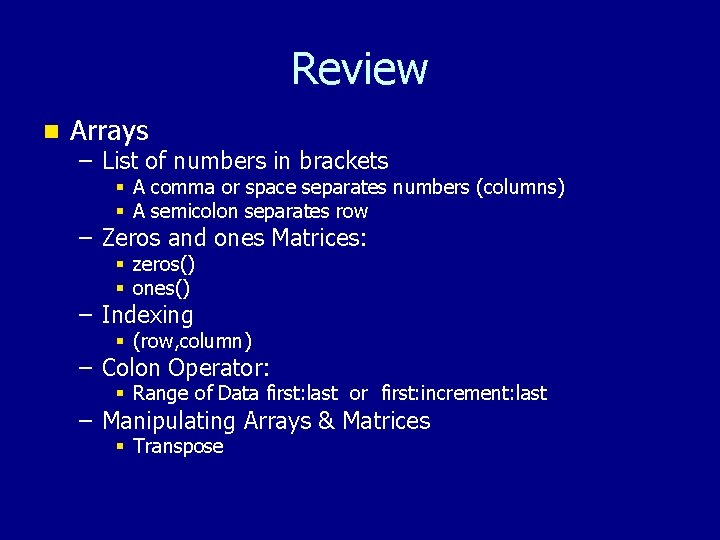Review n Arrays – List of numbers in brackets § A comma or space separates numbers (columns) § A semicolon separates row – Zeros and ones Matrices: § zeros() § ones() – Indexing § (row, column) – Colon Operator: § Range of Data first: last or first: increment: last – Manipulating Arrays & Matrices § Transpose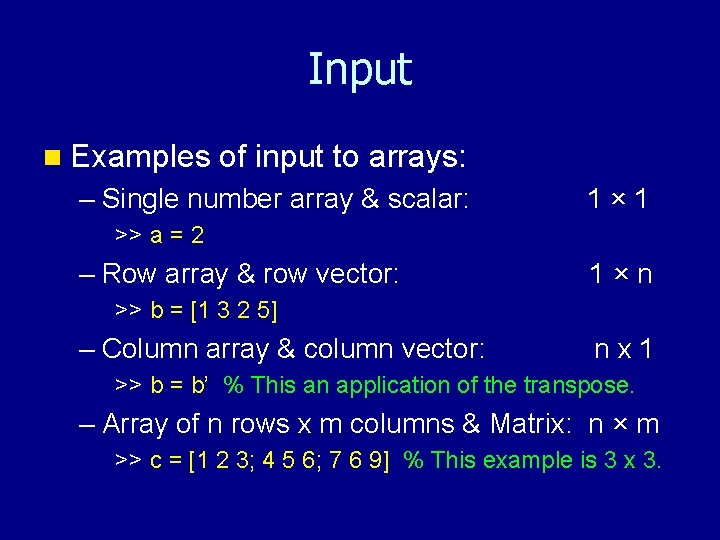Input n Examples of input to arrays: – Single number array & scalar: 1× 1 >> a = 2 – Row array & row vector: 1×n >> b = [1 3 2 5] – Column array & column vector: nx 1 >> b = b’ % This an application of the transpose. – Array of n rows x m columns & Matrix: n × m >> c = [1 2 3; 4 5 6; 7 6 9] % This example is 3 x 3.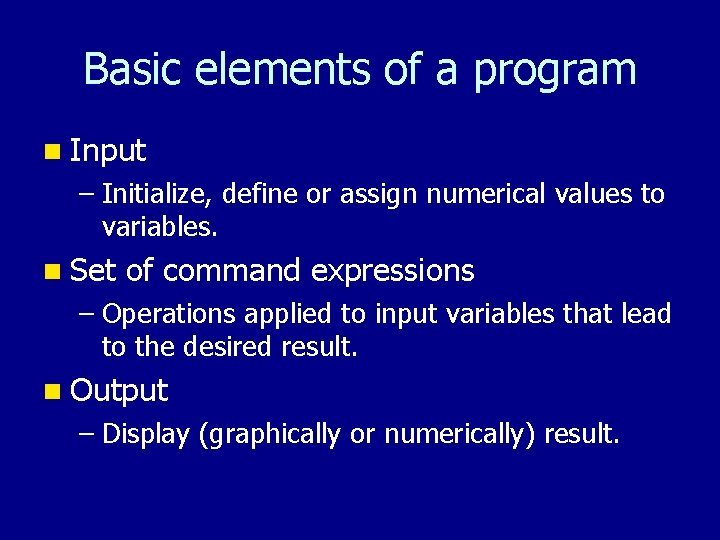Basic elements of a program n Input – Initialize, define or assign numerical values to variables. n Set of command expressions – Operations applied to input variables that lead to the desired result. n Output – Display (graphically or numerically) result.An example of technical computing n Let us consider using the hyperbolic tangent to model a down-hill section of a snowboard or snow ski facility. n Let us first examine the hyperbolic tangent function by executing the command: >> ezplot( ‘tanh(x)’ ) We get the following graph: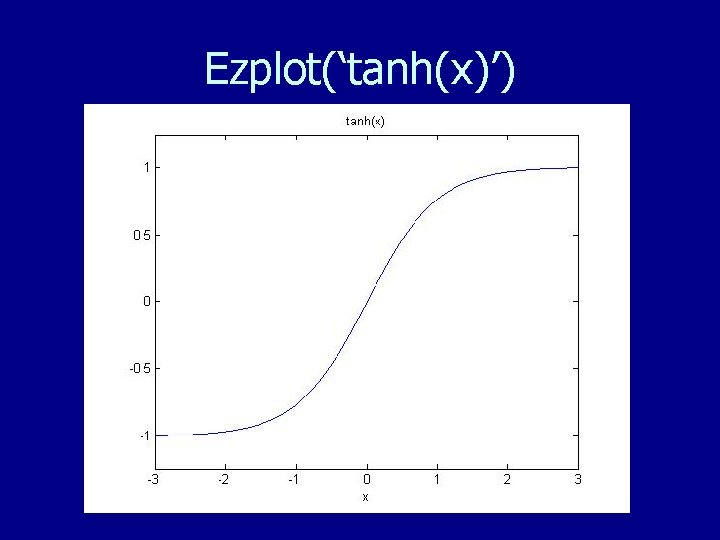Ezplot(‘tanh(x)’)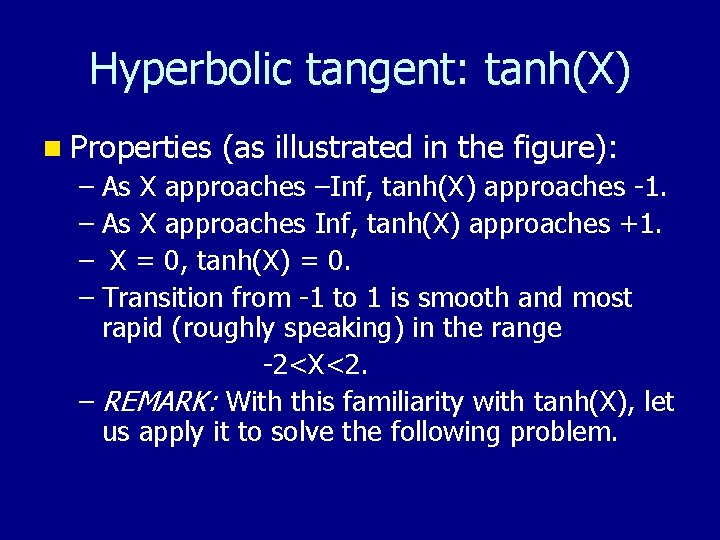Hyperbolic tangent: tanh(X) n Properties (as illustrated in the figure): – As X approaches –Inf, tanh(X) approaches -1. – As X approaches Inf, tanh(X) approaches +1. – X = 0, tanh(X) = 0. – Transition from -1 to 1 is smooth and most rapid (roughly speaking) in the range -2<X<2. – REMARK: With this familiarity with tanh(X), let us apply it to solve the following problem.Problem background n Let us consider the design of a slope that is to be modeled by tanh(X). Consider the range -3<X<3, and assume the slope changes negligibly for |X|>3. n Thus, for – 3 < X < 3, – 1 < tanh(X) < 1. n We want to design a downhill slope such that in 10 meters the hill drops 2 meters. n Thus, as shown next, the following formula is needed: y = 1 – tanh(3*X/5).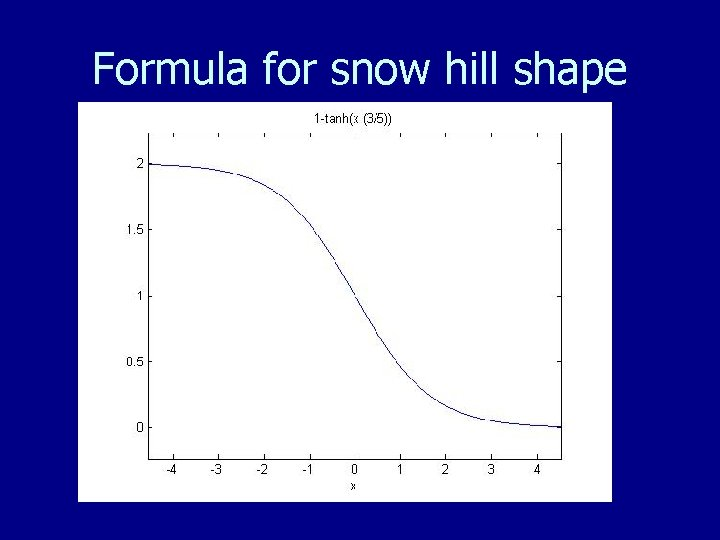Formula for snow hill shape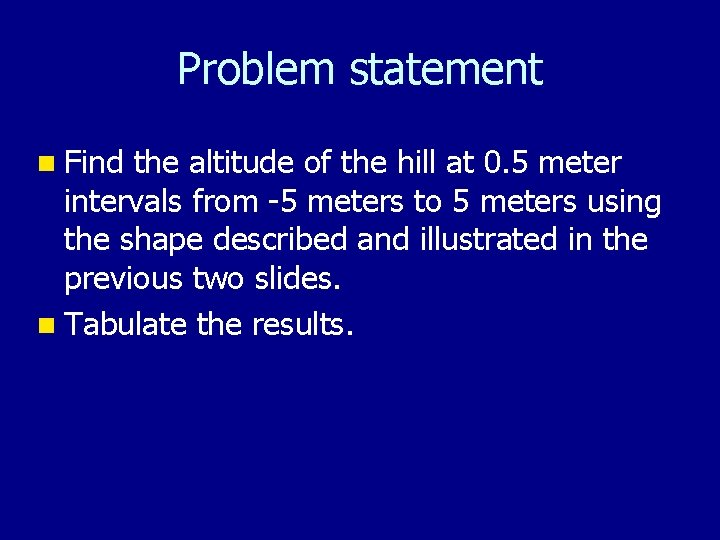Problem statement n Find the altitude of the hill at 0. 5 meter intervals from -5 meters to 5 meters using the shape described and illustrated in the previous two slides. n Tabulate the results.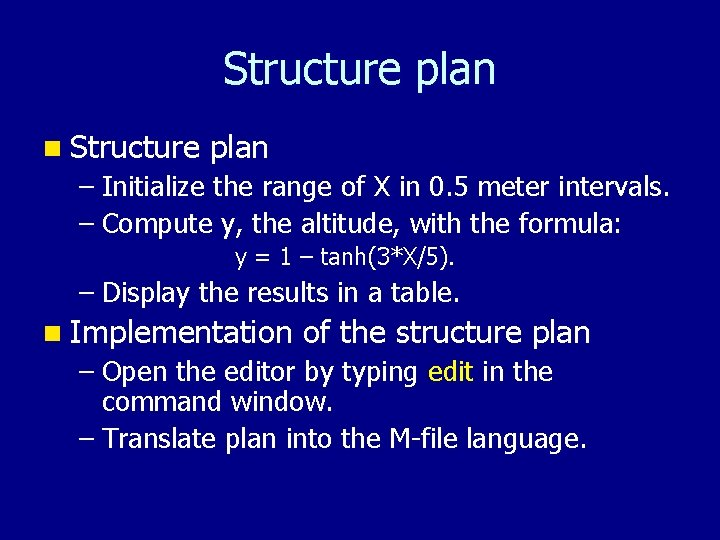Structure plan n Structure plan – Initialize the range of X in 0. 5 meter intervals. – Compute y, the altitude, with the formula: y = 1 – tanh(3*X/5). – Display the results in a table. n Implementation of the structure plan – Open the editor by typing edit in the command window. – Translate plan into the M-file language.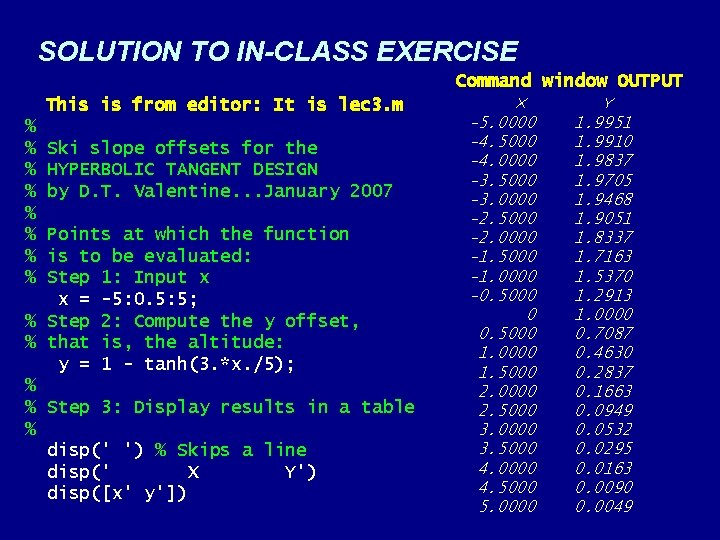SOLUTION TO IN-CLASS EXERCISE Command window OUTPUT This is from editor: It is lec 3. m % % % % Ski slope offsets for the HYPERBOLIC TANGENT DESIGN by D. T. Valentine. . . January 2007 Points at which the function is to be evaluated: Step 1: Input x x = -5: 0. 5: 5; % Step 2: Compute the y offset, % that is, the altitude: y = 1 - tanh(3. *x. /5); % % Step 3: Display results in a table % disp(' ') % Skips a line disp(' X Y') disp([x' y']) X -5. 0000 -4. 5000 -4. 0000 -3. 5000 -3. 0000 -2. 5000 -2. 0000 -1. 5000 -1. 0000 -0. 5000 0 0. 5000 1. 0000 1. 5000 2. 0000 2. 5000 3. 0000 3. 5000 4. 0000 4. 5000 5. 0000 Y 1. 9951 1. 9910 1. 9837 1. 9705 1. 9468 1. 9051 1. 8337 1. 7163 1. 5370 1. 2913 1. 0000 0. 7087 0. 4630 0. 2837 0. 1663 0. 0949 0. 0532 0. 0295 0. 0163 0. 0090 0. 0049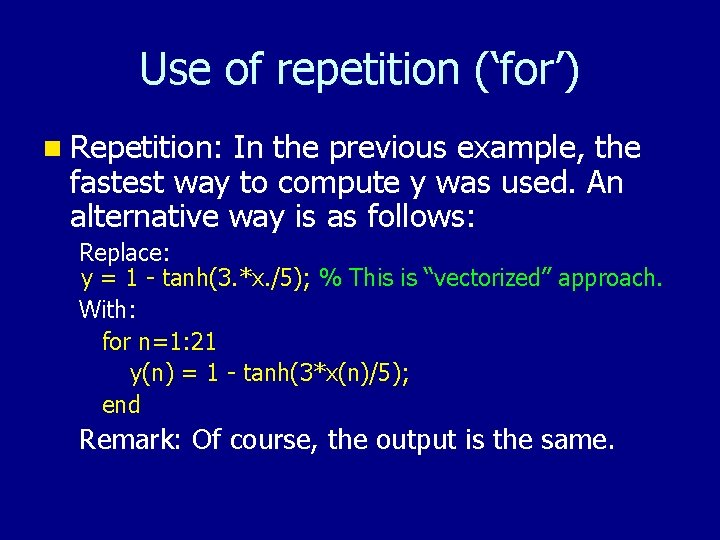Use of repetition (‘for’) n Repetition: In the previous example, the fastest way to compute y was used. An alternative way is as follows: Replace: y = 1 - tanh(3. *x. /5); % This is “vectorized” approach. With: for n=1: 21 y(n) = 1 - tanh(3*x(n)/5); end Remark: Of course, the output is the same.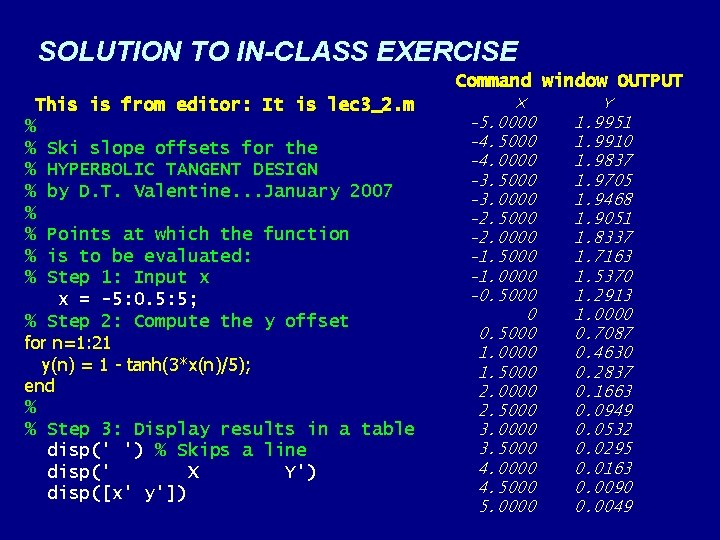SOLUTION TO IN-CLASS EXERCISE Command window OUTPUT This is from editor: It is lec 3_2. m % % Ski slope offsets for the % HYPERBOLIC TANGENT DESIGN % by D. T. Valentine. . . January 2007 % % Points at which the function % is to be evaluated: % Step 1: Input x x = -5: 0. 5: 5; % Step 2: Compute the y offset for n=1: 21 y(n) = 1 - tanh(3*x(n)/5); end % % Step 3: Display results in a table disp(' ') % Skips a line disp(' X Y') disp([x' y']) X -5. 0000 -4. 5000 -4. 0000 -3. 5000 -3. 0000 -2. 5000 -2. 0000 -1. 5000 -1. 0000 -0. 5000 0 0. 5000 1. 0000 1. 5000 2. 0000 2. 5000 3. 0000 3. 5000 4. 0000 4. 5000 5. 0000 Y 1. 9951 1. 9910 1. 9837 1. 9705 1. 9468 1. 9051 1. 8337 1. 7163 1. 5370 1. 2913 1. 0000 0. 7087 0. 4630 0. 2837 0. 1663 0. 0949 0. 0532 0. 0295 0. 0163 0. 0090 0. 0049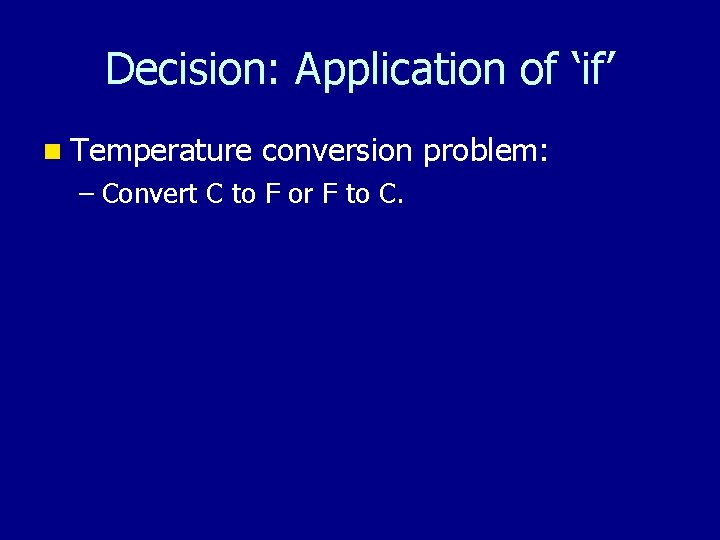Decision: Application of ‘if’ n Temperature conversion problem: – Convert C to F or F to C.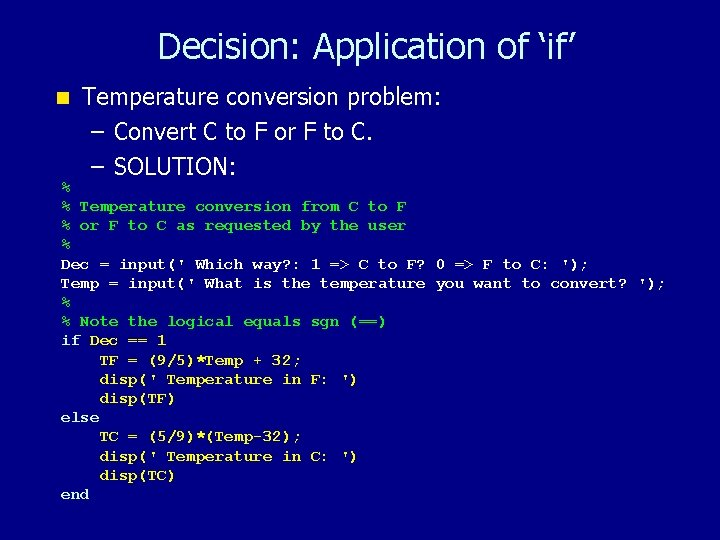Decision: Application of ‘if’ n Temperature conversion problem: – Convert C to F or F to C. – SOLUTION: % % Temperature conversion from C to F % or F to C as requested by the user % Dec = input(' Which way? : 1 => C to F? 0 => F to C: '); Temp = input(' What is the temperature you want to convert? '); % % Note the logical equals sgn (==) if Dec == 1 TF = (9/5)*Temp + 32; disp(' Temperature in F: ') disp(TF) else TC = (5/9)*(Temp-32); disp(' Temperature in C: ') disp(TC) end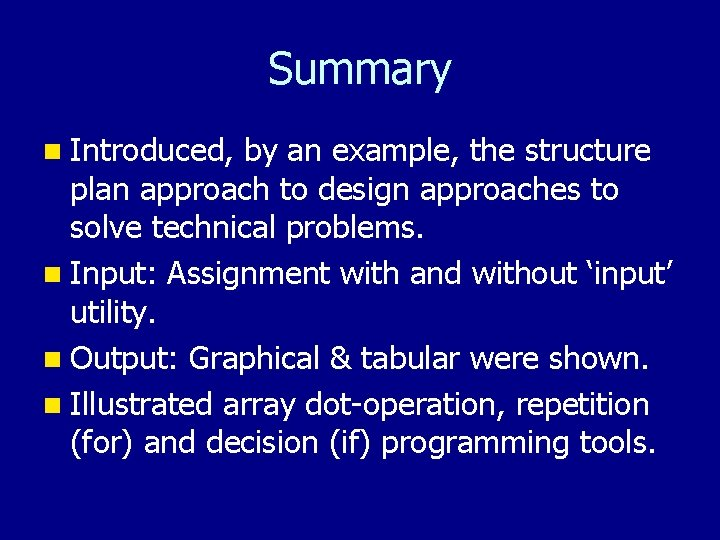Summary n Introduced, by an example, the structure plan approach to design approaches to solve technical problems. n Input: Assignment with and without ‘input’ utility. n Output: Graphical & tabular were shown. n Illustrated array dot-operation, repetition (for) and decision (if) programming tools.